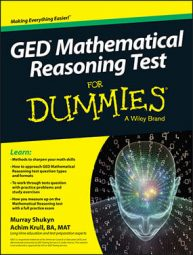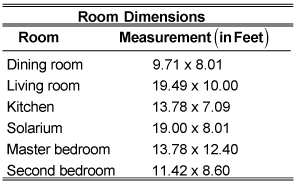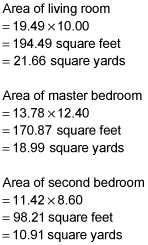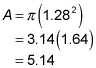##### GED Mathematical Reasoning Test For DummiesSeveral questions on the GED Mathematical Reasoning test require you to know how to calculate the perimeter (or diameter) and area of two-dimensional objects, including rectangles, triangles, and circles.

You'll have a formula sheet at your fingertips, but you'll still have to know how to use the formulas, as well as how to figure out what the question is asking. The following practice questions are similar to what you'll run across on the test.

## Practice questions

1. Matilda has bought a new apartment and wants to carpet the living room, master bedroom, and second bedroom. She records the following measurements:If she budgets \$27.50 per square yard for carpeting and installation, how much should she budget for these rooms?

A. \$1,340

B. \$12,870

C. \$1,430

D. \$1,287

2. Sam is making a circular carpet, using small pieces of carpeting glued onto a backing. If he wants a carpet that is 7 feet 8 inches across, including a 2-inch fringe all around the carpet backing, how many square yards of backing does he need to cover the carpet, rounded to the second decimal place?

E. 45.9

F. 5.10

G. 5.15

H. 5.14

## Answers and explanations

1. C.

She should budget \$1,430 for all the rooms. To calculate this amount, start by figuring out the total area:She would need a total of 21.66 + 18.99 + 10.91 = 51.56 square yards of carpeting.

Because carpeting is sold in even square yards, rounded up, Matilda would have to budget 52 square yards of carpeting at \$27.50 per square yard = \$1,430.

2. F. 5.10 square yards.

The diameter of the backing is 7 feet 8 inches, or 7.67 feet. Therefore, the radius is 3.84 feet, or 1.28 yards.

Substituting in the formula for the area of a circle:square yards, or 5.10 square yards rounded to the second decimal point.